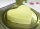# Ratio + expression of a variable from the formula - math problems

#### Number of problems found: 109

• Coils of transformerThe primary coil of the transformer has 400 turns, a current of 1.5 A passes through it and is connected to a voltage of 220 V. For the secondary coil, find the voltage, current, and a number of turns if the transformation ratio k = 0.1.
• Base of prismThe base of the perpendicular prism is a rectangular triangle whose legs length are at a 3: 4 ratio. The height of the prism is 2cm smaller than the larger base leg. Determine the volume of the prism if its surface is 468 cm2.
• SSA and geometryThe distance between the points P and Q was 356 m measured in the terrain. The PQ line can be seen from the viewer at a viewing angle of 107° 22 '. The observer's distance from P is 271 m. Determine the viewing angle of P and observer.
• Conical bottleWhen a conical bottle rests on its flat base, the water in the bottle is 8 cm from it vertex. When the same conical bottle is turned upside down, the water level is 2 cm from its base. What is the height of the bottle?
• PumpsPump that draws water at velocity 3.5 liters per second water from a construction trench take 35 minutes. a) Find out how many minutes the water would run out of the trench pump that draws 7.4 liters of water per second. b) What is the pumping velocity wo
• Similar trianglesThe triangles ABC and XYZ are similar. Find the missing lengths of the sides of the triangles. a) a = 5 cm b = 8 cm x = 7.5 cm z = 9 cm b) a = 9 cm c = 12 cm y = 10 cm z = 8 cm c) b = 4 cm c = 8 cm x = 4.5 cm z = 6 cm
• Sugar productionFrom 1 ton of beet, 150 kg of sugar is produced. To clean 1 ton of sugar, 450 kg of lime is consumed. Calculate how many kgs of lime is consumed when processing 1 ton of sugar beet?
• Squares above sidesTwo squares are constructed on two sides of the ABC triangle. The square area above the BC side is 25 cm2. The height vc to the side AB is 3 cm long. The heel P of height vc divides the AB side in a 2: 1 ratio. The AC side is longer than the BC side. Calc
• Equilateral coneWe pour so much water into a container that has the shape of an equilateral cone, the base of which has a radius r = 6 cm, that one-third of the volume of the cone is filled. How high will the water reach if we turn the cone upside down?

We apologize, but in this category are not a lot of examples.
Do you have an interesting mathematical word problem that you can't solve it? Submit a math problem, and we can try to solve it.

We will send a solution to your e-mail address. Solved examples are also published here. Please enter the e-mail correctly and check whether you don't have a full mailbox.

Please do not submit problems from current active competitions such as Mathematical Olympiad, correspondence seminars etc...

Check out our ratio calculator. Ratio - math word problems. Expression of a variable from the formula - math word problems.